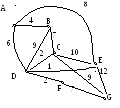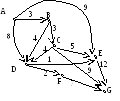# Spring 2003 cse331 Midterm 2 (75 minutes) Sections 1,2,3 Chung

 Date 28.05.2018 Size 80.44 Kb.
Spring 2003 CSE331 Midterm 2 (75 minutes)

Sections 1,2,3 Chung

Name ________________________________________ID______________________

PART I. (15 points.) Multiple choice. No explanation necessary.

1. Suppose we consider a variation of Mergesort in which we divide the list to be sorted into four sublists of equal size, recursively sort each sublist, and then merge the four lists to obtain the final sorted list. What recurrence would be the number of comparisons used by this algorithm in the worst case?

A. T(n) = 2 T(n/2) + n - 1. B. T(n) = 4 T(n/2) + n - 1.

C. T(n) = 2 T(n/4) + n - 1. D. T(n) = 4T(n/4) + n - 1.

E. T(n) = 4T(n/4) + n2.

1. In class we mentioned that Mergesort and Quicksort could be viewed as applications of a technique called Divide-and-Conquer. In this technique we solve a problem by

1. dividing it into subproblems,

2. recursively solving the subproblems, and then

3. combining the solutions of the subproblems to get a solution to the original problem.

(i) Outside of the time spent in recursive calls, where did Quicksort do its comparisons of keys?

1. In stage (a).

2. In stage (c).

3. About half in stage (a), and the other half in stage (c).

4. Neither stage (a) nor stage (c) required any comparisons of keys.

(ii) Outside of the time spent in recursive calls, where did Mergesort do its comparisons of keys?

1. In stage (a).

2. In stage (c).

3. About half in stage (a), and the other half in stage (c).

4. Neither stage (a) nor stage (c) required any comparisons of keys.

3. Consider the following program. What is the time complexity of the following program?

Long power(int x, int n)

{

if ( n == 1 ) return x;

else {

if ( n % 2 == 0 ) return power(x,n/2)*power(x,n/2) ;

else return power(x,n/2)*power(x,n/2) *x

}

}

A. O(n) B. O(nlogn) C. O(logn) D. O(n2) D. O(2n)

PART II. (25 points) True or false? If true, explain it briefly. If not, provide a counter example.

1. Given a connected undirected graph G, if the weights of all edges are distinct, there is at most one minimum spanning tree.

1. Given a connected undirected graph G, if the weights of all edges are distinct, there is exactly one shortest path from one node to another node.

1. Dijkstra’s algorithm works even when there is a negative edge.

1. Prim’s algorithm works even when there is a negative edge.

1. In hashing of table size 10,000, if there is no deletion of keys and the number of elements are close to 5000, chaining is much better than open addressing (closed hashing).

1. In hashing, as the load factor approaches to 1, the performance of the chaining is better than the closed hashing.

1. For a hash table of size 111, let h1 and h2 be as follows:

h1(x) = 4x+1 mod 105

h2(x) = 7x+1 mod 105

In this case, h1 is better hashing function than h2.

PART III. (30 points)

1. Find the minimum spanning tree using Prim’s algorithms.

3. Below is a set of characters and their relative frequencies of occurrence. Build an optimum Huffman code for this set of characters.

Character: a b c d e f

Frequency: 5 2 6 2 8 4

4. Let z1, z2 be two complex numbers. That is, z1= x1 + iy1, and z2 = x2 + iy2, where x1,x2,y1,y2 are integers, and i = -1 .
Show that z1*z2 can be computed three integer multiplications.

0

1

2

3

4

5

6

7

8

9

5

. Let the hash table size be 10 and

h(x) = 3x + 1 mod 10

C

onsider the following sequence of operations

I

nsert 13

Insert 20

Insert 10

Insert 23

Delete 20

Search 10

Show the contents of hash table for open addressing. Assume that you use linear probing for open addressing. For each operation, how many comparisons with the table entry does it require?

PART IV. (30 points)

Select any three problems and solve them.

Mark clearly which problems should be graded.
1. Find the shortest path spanning tree of the next graph.

(a) Demonstrate clearly how the cost (v) and from(v) are updated after each step.

Fill out the “?” places of step 2, and all places of

Step 2-6.

Step

Selected node

## A

B

C

D

E

F

G

Cost

From

Cost

From

Cost

From

Cost

From

Cost

From

Cost

From

Cost

From

0

A

3

A

-

-

8

A

9

A

-

-

-

-

1

B

0

3

A

B

?

?

B

9

A

-

-

-

-

2

3

4

5

6

(b) What is the shortest path from A to E.

(c) What is the shortest path from A to G.

1. Write a pseudo code of FindMed (A, n) which finds the median of an array A [1..n].

Note that A is not a sorted array. Your algorithm must be O(n) time in average.
You may use Partition(A, i, j), where Partition(A, i, j) returns m, and partitions the list A[i..j] into two parts A[i .. m] and A[m+1 .. j] such that every element of A[i..m] is smaller than or equal to the elements of A[m+1..j].
For example, if A[1..7] = {1,4,6,5,3,7,2}, one result of Partition (A,2,7) is,

it returns 3, with updated A={1,2, 3,5,6,7,4}

Note that the result of Partition(A, i, j) depends on how we implement it and the pivot element picked, but it is not the concern of us for the following problem. Assume that partition will take O(n) time for a list of n elements.

The number of lines should be smaller than 12 lines.

What is the average case complexity of the above program?
Let T(n) be the worst case time complexity of the above algorithm.

What is the recurrence equation describing the T(n)?

What is the worst case complexity of the Find?

3. Consider the following problem.

## Job Scheduling with maximum profit

Instance: Jobs {J1,…,Jn}, with their profits {p1,…,pn}, and deadline {d1,…,dn}.

Objective: Schedule jobs to maximize the total profit. Each job has a unit execution time. Job scheduled within deadline gets its profit, but cannot get profit if it does not end within deadline.
Demonstrate how greedy algorithm works with the following example.

Example: Jobs with deadline and profits
Job J1 J2 J3 J4 J5 J6 J7

Profit 6 2 1 4 3 5 7

Deadline 4 2 5 2 3 1 4

4.The coin-changing problem In a Whacky country, there are k types of coins with their values x1, x2, ..., xk cents.

Given y cents for the change, you would like to get the minimum number of changes.

For the demonstration of your algorithm, work with the following example:

Coins: 1cent, 5 cents, 12 cents

Change: 19 cents
What is the greedy algorithm?

Does it give the optimal solution?

Give a counter example of changes and coins where the greedy algorithm does.